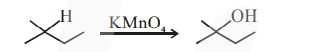# Given below are two statements :Question:

Given below are two statements :

Statement-I : 2-methylbutane on oxidation with $\mathrm{KMnO}_{4}$ gives 2-methylbutan-2-ol.

Statement-II : $\mathrm{n}$-alkanes can be easily oxidised to corresponding alcohol with $\mathrm{KMnO}_{4}$.

Choose the correct option :

1. Both statement I and statement II are correct

2. Both statement I and statement II are incorrect

3. Statement I is correct but Statement II is incorrect

4. Statement I is incorrect but Statement II is correct

Correct Option: , 3

Solution:

Alkane are very less reactive, tertiary hydrogen can oxidise to alcohal with $\mathrm{KMnO}_{4}$.2-methyl-butane